# Active matter logic for autonomous microfluidics

## Abstract

Chemically or optically powered active matter plays an increasingly important role in materials design, but its computational potential has yet to be explored systematically. The competition between energy consumption and dissipation imposes stringent physical constraints on the information transport in active flow networks, facilitating global optimization strategies that are not well understood. Here, we combine insights from recent microbial experiments with concepts from lattice-field theory and non-equilibrium statistical mechanics to introduce a generic theoretical framework for active matter logic. Highlighting conceptual differences with classical and quantum computation, we demonstrate how the inherent non-locality of incompressible active flow networks can be utilized to construct universal logical operations, Fredkin gates and memory storage in set–reset latches through the synchronized self-organization of many individual network components. Our work lays the conceptual foundation for developing autonomous microfluidic transport devices driven by bacterial fluids, active liquid crystals or chemically engineered motile colloids.

## Introduction

Active materials1,2 powered by light or chemical sources offer intriguing technological and biomedical potential, from targeted drug delivery3 and microscale reactors4,5 to tissue engineering6 and energy harvesting7,8. An important subgroup of active materials is fluid-based2, encompassing ATP-driven liquid crystals9,10, bromine-fueled squirmer droplets11, Janus particles12,13,14, colloidal rollers15 and microbial suspensions16,17. These systems are central to current microfluidic soft robotics research18 owing to their ability to self-assemble into complex structures12,13,14, spontaneously create unidirectional flows19 and transport microcargos7,20. While much has been learned about the ordering principles of active fluid systems in the last decade2, their intrinsic computational potential has yet to be systematically explored and exploited21,22,23.

The recent discovery of collective bacterial spin states17 suggests that self-organized active flows can be utilized for microfluidic information storage or transport. Moreover, certain classes of organisms, such as the slime mould Physarum polycephalum24,25, use fluid-mediated computation strategies to solve complex optimization problems, but the decentralized algorithms at work have yet to be deciphered. Microfluidic technology has been successfully employed to perform universal Boolean computation through sub-millimetre bubbles26,27, enabling the logical control of chemical micro-reactors in lab-on-a-chip devices. However, bubble logic requires an externally applied pressure difference26,27, analogous to an applied voltage in a conventional computer. Ferrofluid droplet computation28 similarly depends on an external rotating magnetic field ‘clock’. By contrast, active liquids can flow spontaneously9,10,11,19, while still undergoing complex global topological interactions29. This makes active fluids a promising candidate for the implementation of autonomous computation schemes to drive microfluidic reaction, mixing and transport devices and uncover algorithmic principles underlying decentralized decision-making in Physarum24,25 and other organisms.

The computational power of any physical or biological system is limited by design choices30 and thermodynamic constraints31. Classical Turing-type machines30 sequentially perform localized binary operations, while being energetically limited through Landauer’s principle32. Quantum computers33 exploit nonlocal entanglement but typically require very low operating temperatures to suppress decoherence. Neural circuits34 rely on feedback loops that can be expensive to maintain35. DNA computing36,37 exploits parallelism to counter slow processing speeds. The active flow networks (AFNs) considered here operate far from thermal equilibrium and realize a nonlocal computation approach that functions at room temperature by combining global incompressibility with local energy conversion constraints. The balance of energy uptake and dissipation forces a microbial or ATP-driven fluid to travel at a preferred speed along micro-channels19, while fluid incompressibility imposes topological constraints on the flow network dynamics that enable the implementation of logical operations. A compact mathematical description of AFNs is made possible by a recently proposed mapping onto an effective lattice-field theory29. Here, we use this generic framework to construct active matter logic (AML): we implement universal logical operations, reversible gates and memory storage in set–reset (SR) latches through the synchronized action of many individual AFN components. We also evaluate the robustness of AFN-based computation against noise.

## Results

### Input–output active flow network model

To construct networks capable of logical operations, we first define closed incompressible AFNs as previously introduced29 before augmenting with input–output capability. Our mathematical approach towards describing AFNs takes direct guidance from recent experiments19 demonstrating that highly concentrated suspensions of Bacillus subtilis bacteria spontaneously self-organize into stable unidirectional flows when confined in narrow microfluidic channels (of width <50 μm).

Modelling a network of narrow channels filled with dense active matter, let Γ be an oriented graph comprising vertices V and edges E; that is, a graph with every edge assigned an arbitrary directionality. Active flows along the edges of Γ are then given by the vector Φ=(φe) of fluxes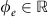along each edge eE, where φe>0 and φe<0 represent flow with and against the orientation of e, respectively. Now, let D=(Dve) be the signed incidence matrix of Γ such that Dve is +1 if e enters v, −1 if e leaves v, and 0 if e is not incident to v. To model spontaneous activity-driven flow along the edges while respecting incompressibility at every vertex, Φ is taken to obey a pseudo-equilibrium model defined by the Hamiltonian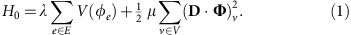The first term, with coupling constant λ, models spontaneous active flow φe→±1 through the double-welled potential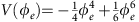, as in Landau-type models. This is subject to the soft incompressibility constraint imposed by the second term with coupling constant μ which, provided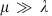, requires the net flux (D·Φ)v at each vertex vV be approximately zero. The energy (1) then yields discrete minima where each edge is either flowing, φe=±1, or in a non-flowing state φe=0 depending on incompressibility-induced topological frustration. Note that a sixth-order flux potential V is necessary to avoid an unphysical hidden symmetry of quartics and guarantee physically realistic19 discrete minima φe{−1, 0, +1} rather than the continuum of fractional states that would result from the interaction of a quartic potential with the quadratic incompressibility constraint29. Other polynomial potentials not sharing the hidden symmetry of quartics could be used as well and yield results similar to those discussed below.

Closed AFNs can be expanded to implement input–output capability by allowing non-zero flux through special vertices while preserving incompressibility in the bulk, distinct from conventional neural networks34,38. An input–output AFN has augmented vertex set VΓ comprising bulk vertices V, which correspond to the vertices of closed AFNs, and boundary vertices Γ of degree 1 (that is, incident to exactly one edge). The boundary vertices Γ=ΓinΓout function as flux inputs and outputs: input vertices Γin are constrained to have net outward flux according to the prescribed binary input vector I=(Iv), whereas output vertices Γout remain unconstrained; instead, their net flux Ov=(D·Φ)v defines the output vector O=(Ov). This is achieved through the boundary energy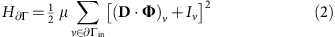constraining (D·Φ)v≈−Iv for all vΓin when, which is added to the bulk energy H0 in equation (1). Finally, we introduce diode edges E+E permitting flow only in their positive direction φe>0, as can be realized through geometric channel patterning39. In particular, we always connect appropriately oriented edges in E+ to the input and output vertices Γ to prevent spurious backflow into or out of the system. This is accounted for through an additional diode energy H+ satisfying H+=∞ if φe<0 for any eE+ and zero otherwise, giving a total energy H=H0+HΓ+H+ for an input–output AFN.

When coupled to an environment that acts as a heat bath, AFNs obey dynamics that are closely related to the Toner–Tu model of polar active fluids40,41. In the absence of complex correlation statistics42, the flow Φ is taken to obey the Langevin equation29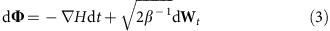with inverse noise strength β, where Wt is a vector of independent unit-variance Brownian processes, resulting in a Boltzmann stationary distribution exp[−βH(Φ)]. Now, in the limit μ→∞, Φ is constrained to the submanifold of incompressible and input-respecting flows—that is, (D·Φ)v=0 for all vV and (D·Φ)v≈−Iv for all vΓin, respectively—on which the components obey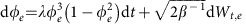. On the other hand, in a narrow channel whose long axis is parallel to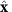, the lowest-order Toner–Tu equation40,41 for the incompressible uniform polarisation field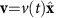reduces to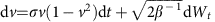for activity strength σ. The usual Toner–Tu term v(1−v2) is simply the beginning of a phenomenological gradient expansion and serves an identical purpose to our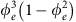which, as discussed above, derives from a potential which can be of any double-welled form beyond simple quartic. Therefore, when many such channels are linked together with flux-conserving boundary conditions in a coarse-grained networked form of an incompressible Toner–Tu model43,44,45, AFN physics arise as a result of the shared Landau theory roots.

AFNs allow us to construct logical circuits in a conceptually different fashion to classical logic. Stable states of an AFN, which are local minima of H, comprise flowing edges with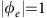and non-flowing edges with φe=0, subject to conservation of flux at every vertex. When Γ is restricted to vertices of degree at most 3, as we will do here, the incompressibility constraint then implies that states must have either zero or two flowing edges incident to every bulk vertex. Stable states therefore comprise vertex-disjoint paths of flowing edges from each active input to distinct outputs and, where possible, closed vertex-disjoint cycles of flowing edges through other internal vertices. The configuration energy H is then proportional to the (negative of the) number of flowing edges, favouring states with greater total flow. Because input–output flows are disjoint, they constrain one another’s allowable locations according to the global topology of Γ. Thus the behaviour with one input active can be changed globally by activating a second input, suggesting that complex operations can be computed by appropriately designed networks. (Note that if a more complex form of V(φe) is employed in equation (1) which does not respect V(±1)<V(0), the situation reverses and states with lesser flow become favoured, in contrast to the spontaneous flow character of active matter. In such a scenario, topologically-rooted flow interactions are less common and our control is more limited.) This is particularly true in the zero-noise limit β→∞ when the only states—the ground states of H—are those with the maximum possible number of edges flowing. It is in this limit that we have the most control in order to create active logic gates, whose ground states at different input choices have output values yielding particular logical operations, as we now show.

### Logic gates

The elementary operations AND (⁁) and OR () can be realized simultaneously as the ground states of a single small active network (Fig. 1a). The network accepts two inputs, X and Y, and its two outputs give XY and X Y. This is achieved through a single cross-input coupling edge and the insertion of simple edges before one output (X Y) to render it energetically favourable to the other (XY) when only one input is active. For example, consider the input state X=0, Y=1. As there are no loops in this network, any ground state must comprise a single connected active flow from Y to one of the two outputs. In this case, there are only two such flow states: one flowing from Y to the X Y output, and one from Y to the XY output. However, the former of these has five edges in a flowing state whereas the latter has only three edges flowing. Thus the former has lower energy than the latter, implying that for this pair of inputs there is only one ground state, whose outputs correctly read X Y=1 and XY=0.

The operation NOT (¬) can also be simply realized (Fig. 1b). Unlike AND/OR, as NOT must output 1 from an input of 0, flux conservation demands an additional power leg permanently fixed at 1; conversely, to output 0 for an input of 1 with power also present, conservation demands two ground legs, whose value is ignored, down which the input and power can be dumped. These concepts are familiar from traditional conservative logic46, wherein logical operations are performed using elements that route unit signals around a circuit while neither creating nor destroying those signals. The axioms of conservative logic also demand one-to-one mapping of input tuples to output tuples, termed reversibility, for all operations, which is not necessarily implied by conservation. However, simultaneously conservative and reversible gates can be constructed in AML, as we will see later.

In classical logic circuits, chaining AND and NOT yields the universal gate NAND which can be used to implement arbitrary Boolean logic. Similar concatenation can be performed in AFNs, provided care is taken to preserve the required ground states. Non-trivial global effects mean that naively combining the networks for AND and NOT in Fig. 1 does not immediately yield NAND: upon merging the output edge for XY in Fig. 1a with the input edge in Fig. 1b, the new path from the X input to the ground legs of the added NOT means the resultant network has two ground states for the input combination X=1, Y=0, one with output 0 and the other with output 1. Nevertheless, active networks can still be exploited to construct NAND in this fashion. The naive concatenation fails because the configuration that should be sending zero input into the appended NOT portion no longer has sufficient energy to retain its unique ground state given the added extra flow path. Therefore, by taking the above construction and inserting an additional edge before what was the X Y output, the path from X to this ignored output is made energetically preferable to the spurious new path from X into the NOT portion when Y=0. This restores the desired ground states (Fig. 1c). Put broadly, if appending a new gate to an output, any additional candidate paths for input states intended to send zero into the appended portion can be disfavoured by upweighting all output and ground legs in the original portion through insertion of extra output-adjacent edges. In practice, such functional stabilization could be achievable by tuning channel geometry19.

Another departure from classical circuits is in the FAN-OUT operation or signal splitter, taking one input and replicating it on two identical outputs. As active network flows are effectively discretized, a signal cannot be copied simply by splicing on another wire; rather, FAN-OUT is itself a powered and grounded active circuit. In fact, as X is output on both ground legs, FAN-OUT is realized simultaneously with NOT in Fig. 1b, akin to the simultaneous realization of AND and OR in Fig. 1a. This circuit can then be appended to any other gate to increase that gate’s fan-out count, provided other output legs are lengthened as necessary in order to preserve the required ground state paths. In general, as in traditional conservative logic46, the ground legs of an operation may output other useful logical expressions that can simplify construction of a larger system. These considerations emphasize how AML is most effective as a top-down, global construction to benefit from the advantage of autonomy inherent in AFNs.

### Environmental noise

Upon taking environmental noise into account, the Langevin equation (3) holds and the density of states is a Boltzmann distribution exp[−βH(Φ)]. AML then becomes probabilistic with a drop-off from perfect accuracy that can be tuned through geometry or microscopic activity9,19. For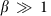and, the order of magnitude of the error—the probability of observing the incorrect result—can be evaluated in terms of the free-edge flow weighting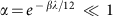by replacing the continuous density of states exp[−βH(Φ)] with that of a discretized system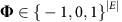and expanding probabilities in α. This estimation shows that while the error in the NAND gate in Fig. 1c is linear in α, the AND/OR network in Fig. 1a is more robust for specific input combinations. In particular, on top of simple mass conservation demanding that AND and OR must be essentially perfect for identical inputs X=Y, AND exhibits error of only O(α3) for X=0, Y=1. This robustness—due to the difference in the number of active edges between the two possible output states—and that of the more sensitive X=1, Y=0 input pair can be further exponentially enhanced by lengthening the upper edge, trading simplicity for accuracy. True operational error can be quantified by numerical evaluation of the marginal distribution p(Ov|I) for the desired output vertex v, as shown for NAND in Fig. 1d by numerical integration of equation (3) at two noise amplitudes (Methods). These results imply that the desired robustness and the noise characteristics of the realization scheme should be taken into account when designing AML systems.

### Memory

Multistable circuits with memory arise naturally within AML as dynamic networks with multiple ground states. A classic set–reset (SR) latch requires at least two logic gates—two NANDs, for instance—and feedback loops between them. In contrast, the global topological feedback inherent in AFNs mean that a memory circuit similar to the SR latch can be constructed very simply (Fig. 2). When S and R are both 0, the two network ground states correspond to outputs Q=0 and Q=1. In the zero noise limit β→∞ these states are stable and the output will not change until one of S or R is changed. On setting S to 1, the flow route from the power leg to ground is cut off and Q immediately sets to 1; upon releasing S, the stable state requiring fewest edge changes (and so nearest in state space) will be favored29 and Q=1 is set. Conversely, setting R to 1 forces the power flow through the ground leg such that releasing R then favors the state with Q=0. Implementing this circuit as a continuous active network obeying the Langevin equation (3)17,29 confirms its memory properties at low noise (Fig. 2c; Methods). Traditional SR latch behaviour, where Q=0 is output immediately on setting R=1, would require a doubly-grounded network capable of dissipating both the power and reset signals.

### Reversible gates

The NAND gate in Fig. 1c is not reversible, since the (X, Y)=(0, 1) and (1, 0) inputs yield identical output and ground leg states: that is, the precise input state cannot be deduced from all readable output data. However, employing closed loops within an AFN, exploiting mutual exclusivity of active flows, allows fully reversible gates to be constructed. For example, a reversible XOR () gate is provided by the two-output CNOT (controlled-NOT) operation familiar to quantum logic33, realized as the ground states of the network in Fig. 3a. This accepts a data input X and a control input C, outputting ¬X if C is 1 and X if C is 0, which is precisely X C, as well as always outputting C, giving a one-to-one mapping of input pairs (X, C) to output pairs (X C, C). The more complex three-input Fredkin or CSWAP gate46, which serves as the fundamental gate of conservative logic46 and is remarkably both reversible and universal, can also be realized in AML (Fig. 3b). In general, mass conservation means that we expect fully reversible computing to be realizable within the AML framework.

## Discussion

Beyond logical operations, finding local energy minima of an AFN can be recast as a Boolean satisfiability problem (SAT) similar to those considered in DNA-based computing36,37. Given a Boolean formula f(x1, …, xn) with logical variables xj{0, 1}, the associated SAT problem asks whether one can find solutions of f(x1, …, xn)=1. To connect this problem with AFNs, suppose that the underlying graph Γ is closed—that is, it has no inputs or outputs (Γ=). To each edge flux φe we associate a Boolean variable xe where xe=1 represents φe=±1 and xe=0 represents φe=0. Incompressibility at a vertex vV then implies the logical condition that an even number of the incident edges eE(v) have xe=1. For example, a bulk degree-3 vertex with incident edge variables x1, x2, x3 has incompressibility condition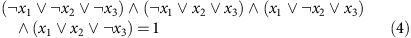in conjunctive normal form. Combining vertices then yields a k-SAT problem—one posed as a large AND of k-term ORs where k is the maximum vertex degree in Γ, whose solutions are potential metastable states of H. If Γ contains no dioded (one-way) edges then these are all energy minima, with multiplicity determined by the number of orientations of the subgraph induced by those eE with xe=1; if there are multiple dioded edges, then some logical states may not be orientable. Input and output vertices simply add further logical conditions: the former force edges to assume 1 or 0 values according to the input vector I, and the dioded edges of the latter reduce to fixing the number of 1-valued output edges to be the number of inputs. In general, many active biological network processes might be fruitfully cast in logical terms: P. polycephalum, for instance, could be viewed as solving a constrained SAT problem to coarsen an initially fine foraging network24,25.

The technology to implement AFN-based logic devices is now becoming available: electrostatically-driven colloids13,15, self-propelled droplets11, polar microfilaments47, artificial extensile nematics9 and microswimmer suspensions17,19,39,48 all present feasible AML realization schemes capable of sustaining microfluidic matter transport. A particularly promising candidate for the experimental implementation of AML schemes could be dense suspensions of Bacillus subtilis bacteria, which spontaneously form robust unidirectional flows in loop-shaped microfluidic channels19 provided the channel diameter is substantially smaller than the preferred bulk vortex size49,50 (70 μm) of an unconfined suspension. Such microbial suspensions are also amenable to rectification through microfluidic ratchets39,51,52, thus enabling unidirectional ‘diode’ edges. With regard to the future, the ideas developed here can be readily expanded to realize more complex design strategies by combining the basic AFNs discussed above with additional external control mechanisms such as optical activation53 or chemical patterning4,54.

To conclude, AFNs present a flexible framework for biologically-rooted computing and autonomous lab-on-a-chip devices. The non-local effects resulting from the interaction of active flows within complex topologies present interesting advantages over classical computing. For instance, eavesdropping detection becomes near-trivial, as any snooping device installed within the network—or even on an ignored ground leg—is likely to fundamentally alter the ground states of the AFN, changing the output behaviour and rendering the intrusion obvious. Fully exploiting the global character of these systems to construct arbitrary computation will require innovative coupling of techniques from statistical physics, control theory and graph theory. Ultimately, this will lend insight into the natural optimization strategies that underly the balance between energy consumption and dissipation constraints present in biological systems.

## Methods

### Numerical integration

Simulations displayed in Figs 1d and 2c were performed by numerically integrating the Langevin equation (3) using the Euler–Maruyama method55. Diode (one-way) edges eE+ were enforced with reflective boundary conditions at φe=0 by setting φe→|φe| at every integration step. Figure 1d uses time step δt=2.5 × 10−3 and includes every 500th point up to t=106, and Fig. 2c uses time step δt=5 × 10−3 and plots every 1000th point. Full Matlab code to perform these simulations is provided as Supplementary Software.

### Network determination

Networks in Figs 1 and 2 possessing ground states obeying the desired logical operations were determined analytically. The CNOT and CSWAP gates in Fig. 3 were found by random search implemented in Mathematica. On generating a random graph Γ with a degree distribution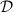, comprising chosen fixed numbers of degree-2 and 3 vertices and as many degree-1 vertices as total inputs and outputs, its ground states for all input combinations were determined by brute force evaluation over all discrete flows. Γ was then deemed a viable candidate if its ground states’ outputs were unique for each input combination and these outputs followed the desired truth table. The numbers of degree-2 and degree-3 vertices inwere varied by trial and error until candidate graphs were found, typically taking on the order of thousands of random graph generations. The chosen candidates were refined by hand to remove unnecessary complexity, and ground states were re-evaluated analytically and further checked against brute-force numerical calculation.

### Data availability

Numerical integration code is provided in Supplementary Software. Any further data available on request to the corresponding author.

How to cite this article: Woodhouse, F. G. & Dunkel, J. Active matter logic for autonomous microfluidics. Nat. Commun. 8, 15169 doi: 10.1038/ncomms15169 (2017).

Publisher’s note: Springer Nature remains neutral with regard to jurisdictional claims in published maps and institutional affiliations.

## References

1. 1

Ge, Q., Qi, H. J. & Dunn, M. L. Active materials by four-dimension printing. Appl. Phys. Lett. 103, 131901 (2013).

2. 2

Marchetti, M. C. et al. Hydrodynamics of soft active matter. Rev. Mod. Phys. 85, 1143 (2013).

3. 3

Din, M. O. et al. Synchronized cycles of bacterial lysis for in vivo delivery. Nature 536, 81–85 (2016).

4. 4

Tompkins, N. et al. Testing Turing’s theory of morphogenesis in chemical cells. Proc. Natl Acad. Sci. USA 111, 4397–4402 (2014).

5. 5

Karzbrun, E., Tayar, A. M., Noireaux, V. & Bar-Ziv, R. H. Programmable on-chip DNA compartments as artificial cells. Science 345, 829–832 (2014).

6. 6

Langer, R. & Vacanti, J. P. Tissue engineering. Science 260, 920–926 (1993).

7. 7

Di Leonardo, R. et al. Bacterial ratchet motors. Proc. Natl Acad. Sci. USA 107, 9541–9545 (2010).

8. 8

Thampi, S. P., Doostmohammadi, A., Shendruk, T. N., Golestanian, R. & Yeomans, J. M. Active micromachines: microfluidics powered by mesoscale turbulence. Sci. Adv. 2, e1501854 (2016).

9. 9

Sanchez, T., Chen, D. T. N., DeCamp, S. J., Heymann, M. & Dogic, Z. Spontaneous motion in hierarchically assembled active matter. Nature 491, 431–434 (2012).

10. 10

Keber, F. C. et al. Topology and dynamics of active nematic vesicles. Science 345, 1135–1139 (2014).

11. 11

Thutupalli, S., Seemann, R. & Herminghaus, S. Swarming behavior of simple model squirmers. New J. Phys. 13, 073021 (2011).

12. 12

Walther, A. & Muller, A. H. E. Janus particles. Soft Matter 4, 663–668 (2008).

13. 13

Yan, J. et al. Reconfiguring active particles by electrostatic imbalance. Nat. Mater. 15, 1095–1099 (2016).

14. 14

Di Leonardo, R. Active colloids: controlled collective motions. Nat. Mater. 15, 1057–1058 (2016).

15. 15

Bricard, A., Caussin, J.-B., Desreumaux, N., Dauchot, O. & Bartolo, D. Emergence of macroscopic directed motion in populations of motile colloids. Nature 503, 95–98 (2013).

16. 16

Sokolov, A. & Aranson, I. S. Physical properties of collective motion in suspensions of bacteria. Phys. Rev. Lett. 109, 248109 (2012).

17. 17

Wioland, H., Woodhouse, F. G., Dunkel, J. & Goldstein, R. E. Ferromagnetic and antiferromagnetic order in bacterial vortex lattices. Nat. Phys. 12, 341–345 (2016).

18. 18

Snezhko, A. & Aranson, I. S. Magnetic manipulation of self-assembled colloidal asters. Nat. Mater. 10, 698–703 (2011).

19. 19

Wioland, H., Lushi, E. & Goldstein, R. E. Directed collective motion of bacteria under channel confinement. New J. Phys. 18, 075002 (2016).

20. 20

Sokolov, A., Apodaca, M. M., Grzybowski, B. A. & Aranson, I. S. Swimming bacteria power microscopic gears. Proc. Natl Acad. Sci. USA 107, 969–974 (2010).

21. 21

Pearce, D. J. G. & Turner, M. S. Emergent behavioural phenotypes of swarming models revealed by mimicking a frustrated anti-ferromagnet. J. R. Soc. Interface 12, 20150520 (2015).

22. 22

Nicolau, D. V., Jr. et al. Parallel computation with molecular-motor-propelled agents in nanofabricated networks. Proc. Natl Acad. Sci. USA 113, 2591–2596 (2016).

23. 23

Wang, A. L. et al. Configurable NOR gate arrays from Belousov-Zhabotinsky micro-droplets. Eur. Phys. J. Spec. Top. 225, 211–227 (2016).

24. 24

Tero, A. et al. Rules for biologically inspired adaptive network design. Science 327, 439–442 (2010).

25. 25

Adamatzky, A. Physarum Machines: Computers from Slime Molds World Scientific (2010).

26. 26

Prakash, M. & Gershenfeld, N. Microfluidic bubble logic. Science 315, 832–835 (2007).

27. 27

Fuerstman, M. J., Garstecki, P. & Whitesides, G. M. Coding/decoding and reversibility of droplet trains in microfluidic networks. Science 315, 828–832 (2007).

28. 28

Katsikis, G., Cybulski, J. S. & Prakash, M. Synchronous universal droplet logic and control. Nat. Phys. 11, 588–596 (2015).

29. 29

Woodhouse, F. G., Forrow, A., Fawcett, J. B. & Dunkel, J. Stochastic cycle selection in active flow networks. Proc. Natl Acad. Sci. USA 113, 8200–8205 (2016).

30. 30

Sipser, M. Introduction to the Theory of Computation 3rd edn Cengage Learning (2013).

31. 31

Landauer, R. Irreversibility and heat generation in the computing process. IBM J. Res. Develop. 5, 183–191 (1961).

32. 32

Berut, A. et al. Experimental verification of Landauer’s principle linking information and thermodynamics. Nature 483, 187–189 (2012).

33. 33

Monroe, C., Meekhof, D. M., King, B. E., Itano, W. M. & Wineland, D. J. Demonstration of a fundamental quantum logic gate. Phys. Rev. Lett. 75, 4714–4717 (1995).

34. 34

Hopfield, J. J. & Tank, D. W. Computing with neural circuits: a model. Science 223, 625–633 (1986).

35. 35

Lestas, I., Vinnicombe, G. & Paulsson, J. Fundamental limits on the suppression of molecular fluctuations. Nature 467, 174–178 (2010).

36. 36

Adleman, L. M. Molecular computation of solutions to combinatorial problems. Science 266, 1021–1024 (1994).

37. 37

Lipton, R. J. DNA solution of hard computational problems. Science 268, 542–545 (1996).

38. 38

Hopfield, J. J. Neural networks and physical systems with emergent collective computational abilities. Proc. Natl Acad. Sci. USA 79, 2554–2558 (1982).

39. 39

Denissenko, P., Kantsler, V., Smith, D. J. & Kirkman-Brown, J. Human spermatozoa migration in microchannels reveals boundary-following navigation. Proc. Natl Acad. Sci. USA 109, 8007–8010 (2012).

40. 40

Toner, J. & Tu, Y. Long-range order in a two-dimensional dynamical XY model: how birds fly together. Phys. Rev. Lett. 75, 4326 (1995).

41. 41

Toner, J. & Tu, Y. Flocks, herds, and schools: a quantitative theory of flocking. Phys. Rev. E 58, 4828 (1998).

42. 42

Schneidman, E., Berry, M. J., Segev, R. & Bialek, W. Weak pairwise correlations imply strongly correlated network states in a neural population. Nature 440, 1007–1012 (2006).

43. 43

Chen, L., Toner, J. & Lee, C. F. Critical phenomenon of the order-disorder transition in incompressible active fluids. New J. Phys. 17, 042002 (2015).

44. 44

Chen, L., Lee, C. F. & Toner, J. Mapping two-dimensional polar active fluids to two-dimensional soap and one-dimensional sandblasting. Nat. Commun. 7, 12215 (2016).

45. 45

Souslov, A., van Zuiden, B. C., Bartolo, D. & Vitelli, V. Topological sound in active-liquid metamaterials. Preprint at http://arxiv.org/abs/1610.06873v2 (2016).

46. 46

Fredkin, E. & Toffoli, T. Conservative logic. Int. J. Theor. Phys. 21, 219–253 (1982).

47. 47

Schaller, V., Weber, C., Semmrich, C., Frey, E. & Bausch, A. R. Polar patterns of driven filaments. Nature 467, 73–77 (2010).

48. 48

Paoluzzi, M., Di Leonardo, R. & Angelani, L. Self-sustained density oscillations of swimming bacteria confined in microchambers. Phys. Rev. Lett. 115, 188303 (2015).

49. 49

Wioland, H., Woodhouse, F. G., Dunkel, J., Kessler, J. O. & Goldstein, R. E. Confinement stabilizes a bacterial suspension into a spiral vortex. Phys. Rev. Lett. 110, 268102 (2013).

50. 50

Dunkel, J. et al. Fluid dynamics of bacterial turbulence. Phys. Rev. Lett. 110, 228102 (2013).

51. 51

Galajda, P., Keymer, J., Chaikin, P. & Austin, R. A wall of funnels concentrates swimming bacteria. J. Bacteriol. 189, 8704–8707 (2007).

52. 52

Kantsler, V., Dunkel, J., Polin, M. & Goldstein, R. E. Ciliary contact interactions dominate surface scattering of swimming eukaryotes. Proc. Natl Acad. Sci. USA 110, 1187–1192 (2013).

53. 53

Palacci, J., Sacanna, S., Steinberg, A. P., Pine, D. J. & Chaikin, P. M. Living crystals of light-activated colloidal surfers. Science 339, 936–940 (2013).

54. 54

Uspal, W. E., Popescu, M. N., Dietrich, S. & Tasinkevych, M. Guiding catalytically active particles with chemically patterned surfaces. Phys. Rev. Lett. 117, 048002 (2016).

55. 55

Higham, D. J. An algorithmic introduction to numerical simulation of stochastic differential equations. SIAM Rev. 43, 525–546 (2001).

## Acknowledgements

This work was supported by Trinity College, Cambridge (F.G.W.), an Alfred P. Sloan Research Fellowship (J.D.), an Edmund F. Kelly Research Award (J.D.), NSF Award CBET-1510768 (J.D.) and a Complex Systems Scholar Award of the James S. McDonnell Foundation (J.D.).

## Author information

Authors

### Contributions

Both authors contributed at all stages of this work.

### Corresponding author

Correspondence to Francis G. Woodhouse.

## Ethics declarations

### Competing interests

The authors declare no competing financial interests.

## Supplementary information

### Supplementary Software

Matlab code to simulate the NAND gate and SR latch with noise by numerical integration of the Langevin equation. (ZIP 4 kb)

## Rights and permissions

Reprints and Permissions

• ### Pattern Engineering of Living Bacterial Colonies Using Meniscus-Driven Fluidic Channels

• Vasily Kantsler
• , Elena Ontañón-McDonald
• , Cansu Kuey
• , Manjari J. Ghanshyam
• , Maria Chiara Roffin
•  & Munehiro Asally

ACS Synthetic Biology (2020)

• ### Spontaneous oscillations and negative-conductance transitions in microfluidic networks

• Daniel J. Case
• , Jean-Régis Angilella

• ### Field generated nematic microflows via backflow mechanism

• Žiga Kos
•  & Miha Ravnik

Scientific Reports (2020)

• ### Reaction processes among self-propelled particles

• Fernando Peruani
•  & Gustavo J. Sibona

Soft Matter (2019)

• ### A dynamic preferred direction model for the self-organization dynamics of bacterial microfluidic pumping

• Daniel Svenšek
• , Harald Pleiner
•  & Helmut R. Brand

Soft Matter (2019)